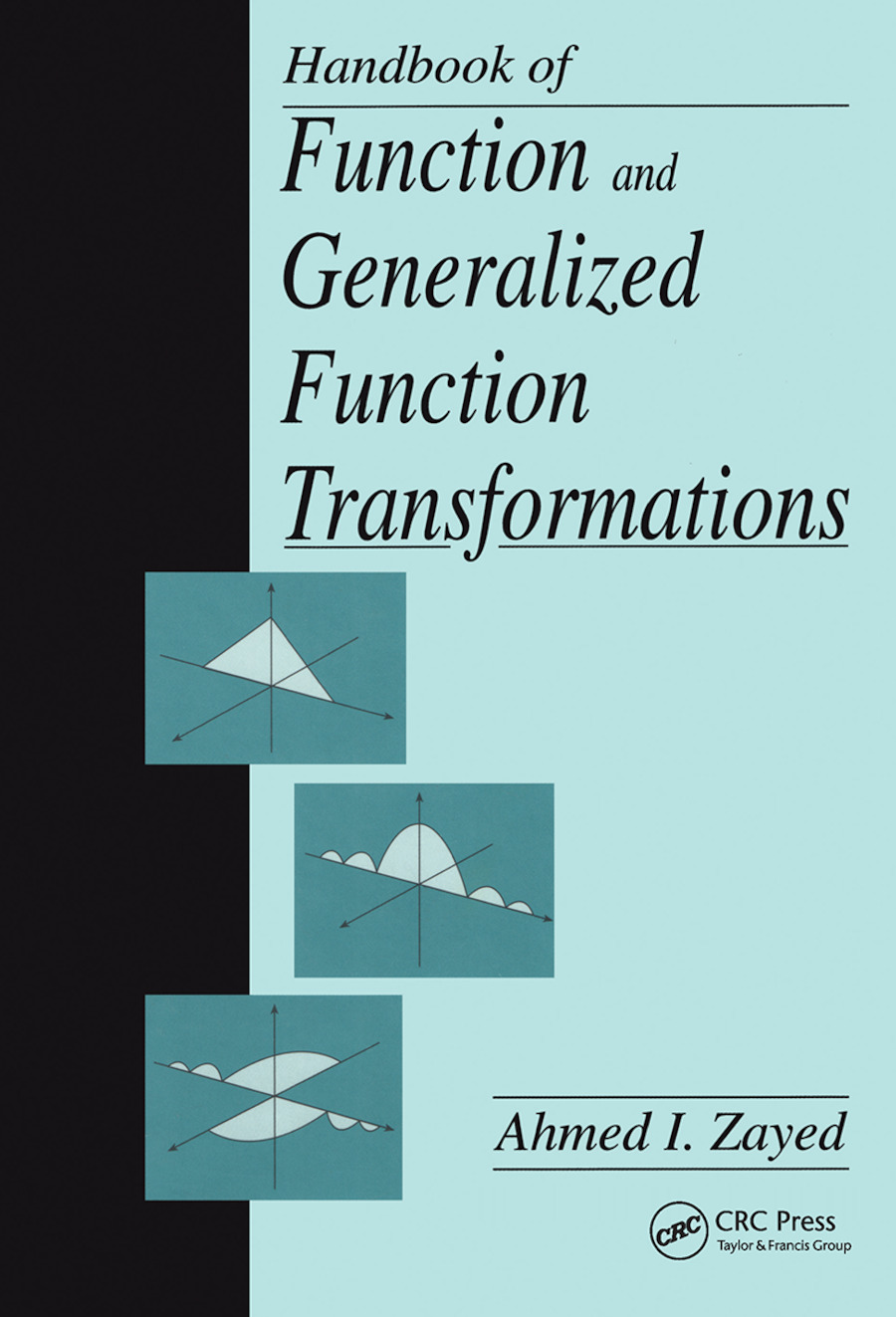Handbook of Function and Generalized Function Transformations

1st Edition

CRC Press

672 pages

Hardback: 9780849378515
pub: 1996-05-15
Currently out of stock
\$240.00
x

FREE Standard Shipping!

Description

Function transformations, which include linear integral transformations, are some of the most important mathematical tools for solving problems in all areas of engineering and the physical sciences. They allow one to quickly solve a problem by breaking it down into a series of smaller, more manageable problems.

The author has compiled the most important and widely used of these function transforms in applied mathematics and electrical engineering. In addition to classical transforms, newer transforms such as wavelets, Zak, and Radon are included.

The book is neither a table of transforms nor a textbook, but it is a source book that provides quick and easy access to the most important properties and formulas of function and generalized function transformations. It is organized for convenient reference, with chapters broken down into the following sections:

Preliminaries

Special Functions

Generalized Functions

Function Transformations

The Laplace Transform

The Two-Sided Laplace Transform

The Borel Transform

The Stieltjes Transform

The Lambert Transform

The Mellin Transform

The Mellin-Type Transform

The Fourier Transform

The Hartley Transform

The Hilbert Transform

The Boas Transform

The Mittag-Leffler Transform

The Convolution Transform

The Weierstrass Transform

The Abel Transform

The Riemann-Liouville and Weyl Fractional Integrals

The Hankel and Hankel-Type Transforms (The I, Y and H-Transforms)

The Hardy Transform

The K(Meijer)-Transform

The Kontorovich-Lebedev Transform

The Mehler-Fock Transform

The Sturm-Liouville-Type Transforms

Miscellaneous Transforms: Kummer, Erdelyi, Hypergeometric, E, and G Transforms

The Bargmann Transform

The Zak Transform

The Gabor Transform

The Ambiguity Transformation and Wigner Distribution

The Wavelet Transform